Next: Spectral estimation Up: SPECTRAL FLUCTUATIONS Previous: Paradox: large n vs.

## An example of the bandwidth/reliability tradeoff

Letting n go to infinity does not take us to the expectation.The problem is, as we increase n, we increase the frequency resolution but not the statistical resolution (i.e., the fluctuation around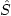). To increase the statistical resolution, we need to simulate ensemble averaging. There are two ways to do this:

1.
Take the sample of n points and break it into k equal-length segments of n/k points each. Compute an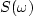for each segment and then average all k of thetogether. The variance of the average spectrum is equal to the variance of each spectrum () divided by the number of segments, and so the fluctuation is substantially reduced.
2.
Formfrom the n-point sample. Replace each of the n/2 independent amplitudes by an average over its k nearest neighbors. This could also be done by tapering the autocorrelation.taper
Figure 10
Spectral smoothing by tapering the autocorrelation.is constant and specified on the top row. Successive rows show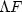increasing while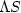decreases. The width of a superimposed box roughly gives, and its height roughly gives.

The second method is illustrated in Figure 10. This figure shows a noise burst of 240 points. Since the signal is even, the burst is effectively 480 points wide, so the autocorrelation is 480 points from center to end: the number of samples will be the same for all cases. The spectrum is very rough. Multiplying the autocorrelation by a triangle function effectively smooths the spectrum by a sinc-squared function, thus reducing the spectral resolution (). Notice thatis equal here to the width of the sinc-squared function, which is inversely proportional to the length of the triangle ().

However, the first taper takes the autocorrelation width from 480 lags to 120 lags. Thus the spectral fluctuationsshould drop by a factor of 2, since the count of terms sk inis reduced to 120 lags. The width of the next weighted autocorrelation width is dropped from 480 to 30 lags. Spectral roughness should consequently drop by another factor of 2. In all cases, the average spectrum is unchanged, since the first lag of the autocorrelations is unchanged. This implies a reduction in the relative spectral fluctuation proportional to the square root of the length of the triangle (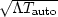).

Our conclusion follows:

The trade-off among resolutions of time, frequency, and spectral amplitude is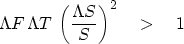(47)Next: Spectral estimation Up: SPECTRAL FLUCTUATIONS Previous: Paradox: large n vs.
Stanford Exploration Project
10/21/1998